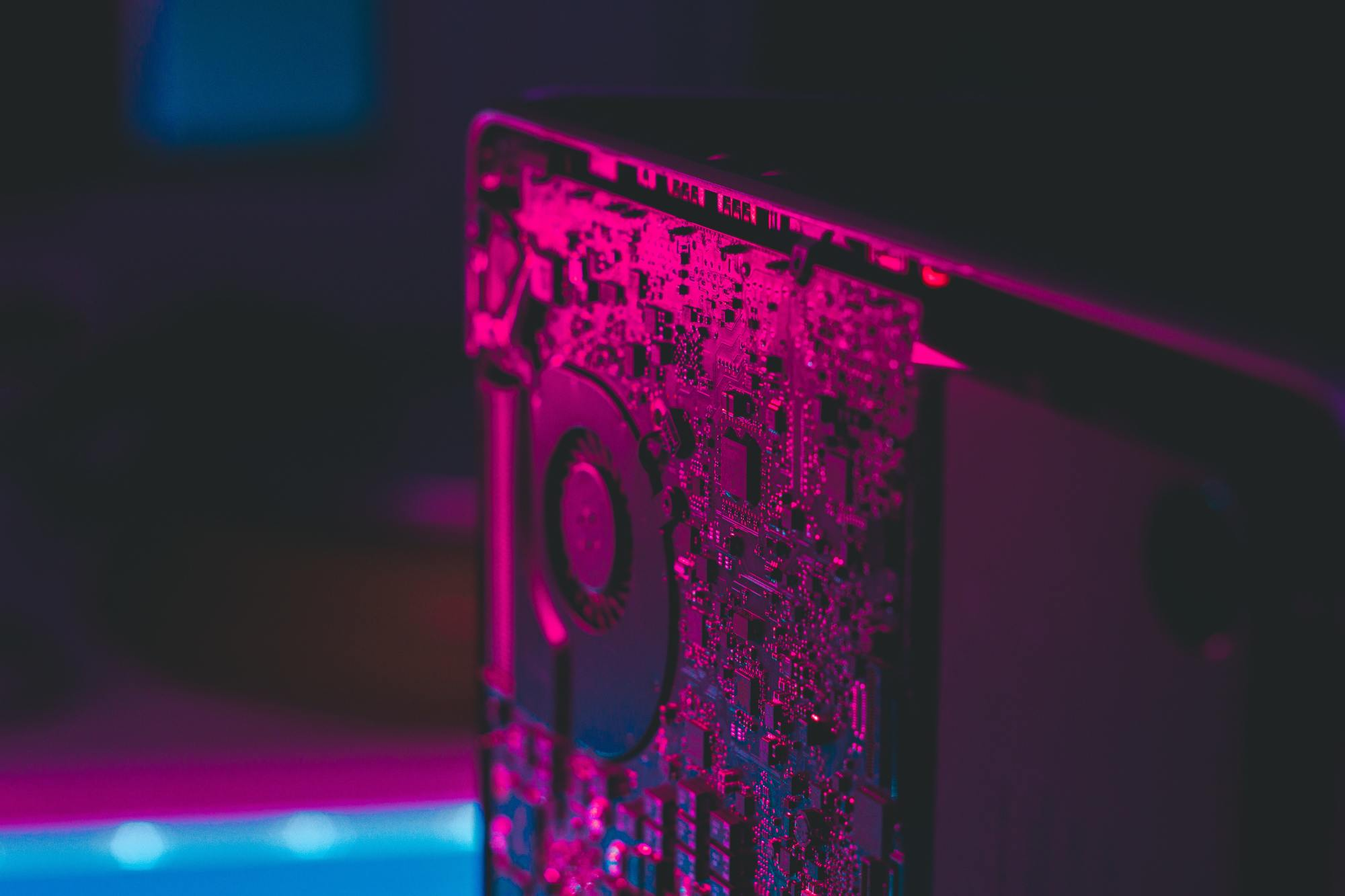#### What’s Quantum Computing and why it matters

At Extendi we are very passionate about technological advancements. Quantum Computing represents a new phase of the computing era, but what is it and how does it work? And most of all, why is it so important? In this article, I’ve tried to answer some of those questions in a simple way so everyone can get a peek into what the future will look like.By Duccio Giovannelli

Founder

10 May 2021What is quantum computing?

Quantum computing is a way to perform calculations that is much different from traditional computing. While traditional computers deal with information in the binary mode where each bit is either turned off or turned on (0-1), quantum computers use so-called qubits. Qubits not only go 0-1 but can also assume a superposition where their state is both 0 and 1 at the same time.

How does it work?

The fact that qubits can assume superposition radically changes the way the computer stores the data and performs calculations. Quantum Computers can perform calculations based on the probability of the state instead of binary calculations.

So let’s say a regular computer has to perform prime factorization of a number. If the number is fairly large the regular computer would need much time to break it down into prime numbers because it would need to go down all paths, one after another, until it finds the right one. If a Quantum Computer was to perform the same task, it would take a fraction of that time because qubits allow quantum computers to go down all the possible paths at once.

Why does quantum computing matter?

Well, try to imagine the world before the invention of calculators. How much time and effort took to perform algebra on very large numbers? A lot! And sometimes it was even impossible. Well, Quantum Computers’ impact can be compared to the introduction of calculators in the modern age.

What are quantum computing applications?

Cryptography, Security, AI, Machine Learning, Healthcare, Banking, Meteorology, Logistics, Finance to name some. Quantum computing’s exponential power will revolutionize a vast range of fields.

If you think about it, all of what the modern world offers does revolve around the principles of mathematics, yet many calculations required for solving problems are way beyond the capability of classical computers (factoring for instance). Quantum computers, on the other hand, will be able to solve these problems, and this will impact almost every sector and aspect of modern existence.

Let’s take the example of cryptography

Modern day cryptography is based on the assumption that current computers don’t have the computational power to break down very large integer numbers into prime numbers. Quantum computers on the other hand can easily break those numbers, thus the code. Quantum-based cryptographic will, in the future, be way more secure than traditional cryptography against quantum hacking.

What should you take from this?

Quantum computing sounds amazingly futuristic and will change the world, yet we are still very far from achieving useful quantum supremacy (a state where quantum computers can beat traditional computers to any task).

Since quantum computers are very expensive to build and operate (and will remain so for long), hopefully, quantum computing power will become available widely through the cloud, and in our future, we will be able to use it for very practical activities like for example finding the fastest path on a map.

Share